# Cross validation assessment of PC models¶

Once a polynomial response surface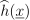of the original numerical model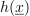has been built up, it is crucial to estimate the approximation error, i.e. the discrepancy between the response surface and the true model response in terms of a suitable norm such as the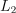-norm: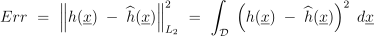where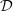denotes the support of the input parameters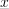. It is worth emphasizing that one tends to overestimate the performance of a response surface by training and evaluating it on the same data set. For instance, the model might fail to predict on new data whereas the validation on the training data yields satisfactory performance. To avoid such issue, it is important that the model error assessment is conducted on a data set which is independent of the training sample. However, any new model evaluation may be time- and memory-consuming. Therefore, error estimates which are only based on already performed computer experiments are of interest. In this context, the so-called cross validation techniques are utilized to obtain reliable performance assessment of the response surface without additional model evaluations.

Any cross-validation scheme consists in dividing the data sample (i.e. the experimental design) into two sub-samples that are independently and identically distributed. A metamodelis built from one sub-sample, i.e. the training set, and its performance is assessed by comparing its predictions to the other subset, i.e. the test set. A single split will lead to a validation estimate. When several splits are conducted, the cross-validation error estimate is obtained by averaging over the splits.

K-fold cross-validation error estimate

The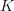-fold cross-validation technique relies on splitting the data set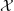intomutually exclusive sub-samples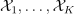of approximately equal size. A sub-sample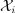,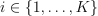is set aside, then the response surface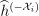is built on the remaining sub-samples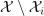. The approximation error is estimated on the set-aside sample: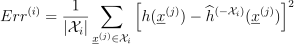in which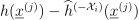is the predicted residual defined as the difference between the evaluation of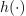and the prediction with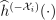at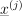in the sub-samplewhose cardinality is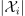.

The approximation errors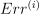are estimated with each of the sub-samples,being used as the validation set whereas the remaining sub-samplesbeing used for training. Finally the-fold cross-validation error estimate is obtained as the average: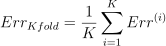As described above, the-fold error estimate can be obtained with a single split of the dataintofolds. It is worth noting that one can repeat the cross-validation multiple times using different divisions into folds to obtain better Monte Carlo estimate. This comes obviously with an additional computational cost.

Classical leave-one-out error estimate

The leave-one-out (LOO) cross-validation is a special case of-fold cross-validation where the number of foldsis chosen equal to the cardinality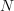of the experimental design. It is recalled that a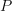-term polynomial approximation of the model response reads: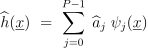where the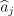’s are estimates of the coefficients obtained by a specific method, e.g. least squares.

Let us denote by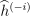the approximation that has been built from the experimental design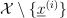with the-th observation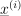being set aside. The predicted residual is defined as the difference between the model evaluation atand its prediction based on:

(1)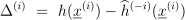By repeating this process for all observations in the experimental design, one obtains the predicted residuals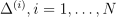. Finally, the LOO error is estimated as follows:

(2)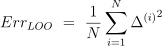Due to the linear-in-parameters form of the polynomial chaos expansion, the quantity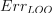may be computed without performing further regression calculations when the PC coefficients have been estimated using the entire experimental design. Indeed, the predicted residuals can be obtained analytically as follows:

(3)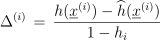where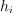is the-th diagonal term of the matrix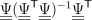with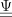being the information matrix:

(4)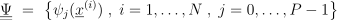In practice, one often computes the following normalized LOO error:

(5)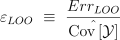where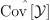denotes the empirical covariance of the response sample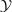:

(6)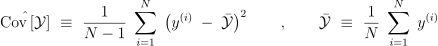Corrected leave-one-out error estimate

A penalized variant of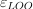may be used in order to increase its robustness with respect to overfitting, i.e. to penalize a large number of terms in the PC expansion compared to the size of the experimental design: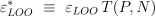The penalty factor is defined by: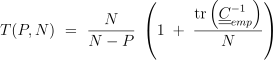where:

(7)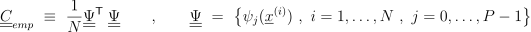Leave-one-out cross validation is also known as jackknife in statistics.